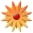# Interpret the equation

Page Views: 1675

Email This Lesson Plan to Me
Overall Rating:(5.0 stars, 1 ratings)

 Keywords: 8th grade math, Slope, y-intercept Subject(s): Algebra, Math Grade 8 NETS-S Standard: Creativity and InnovationCommunication and CollaborationResearch and Information FluencyCritical Thinking, Problem Solving, and Decision MakingDigital CitizenshipTechnology Operations and ConceptsView Full Text of Standards School: Scoggins Middle School, Dallas, GA Planned By: angela bailey Original Author: angela bailey, Dallas
MCC8.F.3 Interpret the equation y = mx + b as defining a linear function, whose graph is a straight line; give examples of functions that are not linear.
(Summative Assessment) Everything is due no later than Friday Feb 28
Grades will be taken from each station/objective
2 Teachers Classroom (3day lesson plan)
Baiey-Objective 1: Students will analysis what role the m (slope) and b(y-intercept) plays in the equation-y=m x+ b
Bailey-Objective 2: Student will select, justify, and apply an appropriate technique to graph linear functions in two variables. Techniques will include slope intercept, x- and y-intercepts, graphing by transformation, and the use of the graphing
Calculator
ESEP Teacher-Objective 1: Students will use their knowledge of coordinate planes to plot order pairs. (Jones has their activities)
ESEP Teacher -Objective 2: Students will apply their knowledge of ordered pairs to calculate the x and y intercept of an equation. (Jones has their activities)
(I DO)Opening/Engage (Use one of the following, coach book or computer, to define the vocabulary words listed below
Introduce the lesson�s vocabulary (Review)
a. Slope
b. Y-intercept
c. Coordinates
d. X-axis>e. Y-axis
Students click the following link to define the words listed above. http://www.nova.edu/~ab1391/home_page.htm .Once you are login to the web page, click resources and scroll down to math dictionary.
Day 1:
(WE DO)Work-time/Explore
Station Rotation: Groups of three
Station 1: Determine the x and y intercept of a line (review)
Students will create a linear equation by standing on the large coordinate grid. One student will stand on a point on the x-axis and one student will stand on a point on the y-axis. The other member will take a yard stick and connect the two points and write down the two order pairs. Calculate the following:
a. Slope
b. X-intercept
c. Equation
d. Sketcha graph using http://www.nova.edu/~ab1391/home_page.htm click on resources and the graphing calculator. Type your equation into the graphing calculator and click graph. Sketch your graph from the information in the calculator.
(Differentiation)If you need additional assistance click the following link http://www.nova.edu/~ab1391/home_page.htm and resources and Algebra 1 Online-modules-linear equations-lesson-3 x and y intercepts-video finding x and y intercept. Given a graph identify the x and y intercept.
Station 2: using http://www.nova.edu/~ab1391/home_page.htm click resources-Algebra 1 Online �modules-linear equations-Activity: Project Graduation Intercept Cards (pdf) students will be given a deck of x and y-intercept cards.
Game: The cards should be shuffled and placed face up on a flat surface. The objective is to match the x-intercept, y-intercept, equation, and graph of each function. Students should determine the order of play. On an individual�s turn, a student should pick the card with the equation of a line and match it to cards showing the x-intercept, y-intercept, or graph. The next student will pick a card that also applies to that same function. Continue play until all cards are matched to their equation.

(Differentiation for Station 2)
a. Teacher will set up the equation for the students and they will calculate the second part of each problem.
b. Teacher will review with the students how to set up a table and have them apply prior knowledge to write the equation.

Station 3: Complete the following story problems and;
a. analyze the slope and the y-intercept
b. Graph your equation on the graphing calculator used in other stations
c. Create a Chunk Paragraph from your data
Problems:
1. The cost of a school banquet is \$95 plus \$15 for each person attending. Write an equation that gives total cost as a function of the number of people attending. What is the cost for 77 people?
2. Billy plans to paint baskets. The paint costs \$14.50. The baskets cost \$7.25 each. Write an equation that gives the total cost as a function of the number of baskets made. Determine the cost of four baskets.

(Differentiation for Station 3)
a. Teacher will set up the equation for the students and they will calculate the second part of each problem.
b. Teacher will review with the students how to set up a table and have them apply prior knowledge to write the equation.
c. Teacher will give the graphic organizer to students to assess them in writing a chunk paragraph.

Students will rotate through the stations (3 days) until all work is completed. (One station a day)

Closing/Explain
Using http://coe.jmu.edu/learningtoolbox/cornellnotes.html write a summary of each station evaluating what you�ve learned.

 Comments I teach math support and student of all abilities are in my classroom. This lesson plan worked very well for all my students. Cross-Curriculum Ideas Using vocabulary to complete the "Chunk Writing" section could be integrated into the Language Arts classes. Links: "Link to my webpage""Link to the intercept cards""Link to Cornell notes""Link to online graphing calculator" Materials: Graphing, Middle School, Printers, Flash/USB Drives, Computer Accessories, Keyboards, Headsets, Middle, Dyslexia